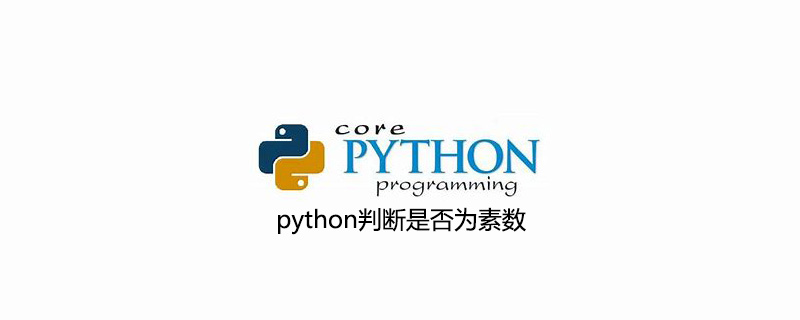• python判断素数函数
千次阅读
2020-11-20 22:24:39

来看这一种判断素数（质数）的函数：

form math import sart

def is_prime(n):

if n==1:

return False

for i in range(2, int(sqrt(n) + 1)):

if n % i == 0:

return False

return True

看起来，这是一种比较优秀的方法了，因为通过sqrt()函数减少了开方级的计算量。

再来看：

def is_prime(number):

if number > 1:

if number == 2:

return True

if number % 2 == 0:

return False

for current in range(3, int(math.sqrt(number) + 1), 2):

if number % current == 0:

return False

return True

return False

咋一看，这一次的代码看起来更多。但是，计算量却又在原来的基础上又几乎减少一半。高明之处就在这一句：if number % 2 == 0:，其实这一句就一部将2以及所有合数因子给排除掉了，所以在这一句range(3, int(math.sqrt(number) + 1), 2)中，直接从3起步，步长为2.在range()函数产生的序列是[3,5,7,9,...],比原来由range(2, int(sqrt(n) + 1))产生的[2,3,4,5,6,...]少了合数的部分。

更多相关内容
• ## python判断素数

万次阅读 多人点赞 2021-02-24 20:05:38
想要判断一个素数，首先要进行一个特殊判断，因为<2的数都不是素数： x = int(input()) if x<2: print("f") 接下来，我们要进行大于2的数的判断。方法就是：用数x对从2到x平方根依次取模，如果结果为0，说明...

想要判断一个素数，首先要进行一个特殊判断，因为<2的数都不是素数：

x = int(input())
if x<2:
print("f")


接下来，我们要进行大于2的数的判断。方法就是：用数x对从2到x平方根依次取模，如果结果为0，说明x不是质数，如果一遍判断下来取模结果都不为0，则说明x为素数

import math
x = int(input())
f = 0

for i in range(2,int(math.sqrt(x))):
if x % i == 0:
print("f")
f = 1

if f == 0:
print("t")


两者结合起来，我们可以创造一个用户自定义函数：

import math

def isprime(x):
if x<2:
return "F"

for i in range(2,int(math.sqrt(x))+1):
if x % i == 0:
return "F"

return "T"

x = int(input())
print(isprime(x))


这个函数就可以满足判断素数的问题

展开全文• 1 #运用python的数学函数23 importmath45 defisPrime(n):6 if n

1 #运用python的数学函数

2

3 importmath4

5 defisPrime(n):6 if n <= 1:7 returnFalse8 for i in range(2, int(math.sqrt(n)) + 1):9 if n % i ==0:10 returnFalse11 returnTrue12

13 #单行程序扫描素数

14

15 from math importsqrt16 N = 100

17 [ p for p in range(2, N) if 0 not in [ p% d for d in range(2, int(sqrt(p))+1)] ]18

19

20 #运用python的itertools模块

21

22 from itertools importcount23 defisPrime(n):24 if n <= 1:25 returnFalse26 for i in count(2):27 if i * i >n:28 returnTrue29 if n % i ==0:30 returnFalse31

32 #不使用模块的两种方法

33

34 defisPrime(n):35 if n <= 1:36 returnFalse37 i = 2

38 while i*i <=n:39 if n % i ==0:40 returnFalse41 i += 1

42 returnTrue43

44

45 defisPrime(n):46 if n <= 1:47 returnFalse48 if n == 2:49 returnTrue50 if n % 2 ==0:51 returnFalse52 i = 3

53 while i * i <=n:54 if n % i ==0:55 returnFalse56 i += 2

57 return True

展开全文• 在本篇文章里小编给大家整理了关于python判断素数的方法和代码，需要的朋友们可以学习下。
• 质数（prime number)又称素数。指在一个大于1的自然数中，除了1和此整数自身外，不能被其他自然数整除的数。...总结python脚本判断一个数是否为素数的几种方法：#运用python的数学函数import mathdef isPrime(n):i...质数（prime number)又称素数。指在一个大于1的自然数中，除了1和此整数自身外，不能被其他自然数整除的数。素数在数论中有着很重要的地位。比1大但不是素数的数称为合数。1和0既非素数也非合数。素数是与合数相对立的两个概念，二者构成了数论当中最基础的定义之一。

总结python脚本判断一个数是否为素数的几种方法：

#运用python的数学函数import math

def isPrime(n):

if n <= 1:

return False

for i in range(2, int(math.sqrt(n)) + 1):

if n % i == 0:

return False

return True

#单行程序扫描素数from math import sqrt

N = 100

[ p for p in range(2, N) if 0 not in [ p% d for d in range(2, int(sqrt(p))+1)] ]

#运用python的itertools模块from itertools import count

def isPrime(n): www.2cto.com

if n <= 1:

return False

for i in count(2):

if i * i > n:

return True

if n % i == 0:

return False

#不使用模块的两种方法def isPrime(n):

if n <= 1:

return False

i = 2

while i*i <= n:

if n % i == 0:

return False

i += 1

return True

def isPrime(n):

if n <= 1:

return False

if n == 2:

return True

if n % 2 == 0:

return False

i = 3

while i * i <= n:

if n % i == 0:

return False

i += 2

return True

展开全文• isPrime4()函数功能相同效率不同，可以引入time判断使用的时间 #涉及简单计时http://www.cnblogs.com/didiaoxiaoguai/p/6684094.html strPrime = strPrime + str(j) + " " print(strPrime) #以下为另一写法 def ...
• 代码 不废话，上代码： ... # 不在 6 的倍数两侧的不是素数 if n % 6 != 1 and n % 6 != 5: return False # 在 6 的倍数两侧的不一定是素数 for i in range(5, int(n ** 0.5) + 1, 6): # i 的步长可以放大到 6算法
• Python习题】判断素数函数（保姆级图文+实现代码） 写一个函数isPrime(n)用于判断一个数字n是不是素数，用户输入一个正整数，在一行内输出不大于该数的所有素数，各数后面用一个空格分隔。‪‬‪‬‪‬‪‬‪‬‮...
• python 3判断素数函数 代码： #函数用来判断是否为素数 def isprime(n): #n为2或3，可以直接判断是素数 if n == 2 or n == 3: return True #n可以被2或3整除，可以直接判断不是素数 if n % 2 == 0 or n % 3 ...算法
• 写一个函数isPrime(n)用于判断一个数字n是不是素数，用户输入一个正整数，在一行内输出不大于该数的所有素数，各数后面用一个空格分隔。 函数接口定义： def isPrime(n): 裁判测试程序样例： /* 请在这里...
• 质数又称素数。指在一个大于1的自然数中，除了1和此整数自身外，不能被其他自然数整除的数。素数在数论中有着很重要的地位。比1大但不是素数的数称为合数。1和0既非素数也非合数。质数是与合数相对立的两个概念，...
• 2、编写函数判断素数，输出flag 代码 import math p=eval(input("请输入您要判读是否是素数的数值：")) k=int(math.sqrt(p)) def prime(p): i=2 flag=True while (i<=k and flag==True):算法
• #coding=utf-8 # 输入一个整数n n = int(input()) # 请在此添加代码，对输入的整数进行判断，如果是素数则输出为True，不是素数则输出为False #********** Begin *********# #定义一个函数prime def prime(n): """ ...编程语言
• 素数（质数）是指在大于1的自然数中，除了1和它本身以外不再有其他因数的自然数 第一种就是暴力解法：遍历2-n的值找出是否存在因数 def IsPrime1(num): if num == 2 or num == 3: return 1 else: for i in ...算法
• https://github.com/ixiaoyu-tech判断一个数是否是素数是每一门语言在学习之初都需要去写的一个程序，今天小玉跟大家分享一下我自己用python写的一个关于判断输入的数是否为素数的程序源码。...
• ## python实现素数判断

千次阅读 2022-03-29 12:42:53
题目要求: ①设计较好的人机对话界面，程序中含有提示性的输入输出语句。②能实现循环操作，由操作者输入相关命令来控制是否继续进行...定义判断素数函数 import math #素数判断，素数返回True，合数返回False def
• 素数素数就是只能被1和自己整除的数 求2和100之内的素数 for x in range（2,101): ...判断是否是素数 p=int(input("请输入一个数：")) if p==1: print(p，"不是素数") for i in range(2,p): if(p
• 一、题目要求 二、参考代码 import math def judge(a): if a==1: return False if a==2: return True for i in range(2,int(math.sqrt(a))): if a%i==0: return False return True ... t = int开发语言 后端
• L1-028 判断素数 - 10 分题目要点代码 题目链接. 题目 本题的目标很简单，就是判断一个给定的正整数是否素数。 输入格式： 输入在第一行给出一个正整数N（≤ 10），随后N行，每行给出一个小于2 31 的需要判断的正...算法
• 给定一个数，判断该数是否为质数。首先来看下质数的定义：质数，又称素数，指的是大于1的自然数中，除了1和自身外，无法被其他自然数整除的数。与质数相对的概念是合数。根据定义，我们可以实现如下的is_prime函数。...
• 说明：本程序首先定义一个判断素数并将其输出的函数IsPrime()IsPrime()IsPrime()，函数包含两个参数aaa和bbb，本程序的作用即输出aaa和bbb之间的所有素数。 Python程序实现 #Date:2019-11-12 #Writer:无名十三 def...
• Python入门程序 函数应用（判断素数、递归求n的阶乘、x的n次方、最大最小值、插入排序法） 1.判断素数 #编写函数，判断一个数是否是素数。 def isprime(n): if n==1: return False for i in range(2, n): if n ...
• 写一个函数isPrime(n)用于判断一个数字n是不是素数，用户输入一个正整数，在一行内输出不大于该数的所有素数，各数后面用一个空格分隔。‪‬‪‬‪‬‪‬‪‬‮‬‭‬‫‬‪‬‪‬‪‬‪‬‪‬‮‬‪‬‭‬‪‬‪‬...
• ## python中判断素数的几种方法

万次阅读 多人点赞 2020-12-14 12:22:08
python统计101~200中素数的个数，并且输出所有的素数。 分析：这是一道典型的循环题。首先，我们应该考虑101~200中得每一个都需要判断是否为素数；其次，每一个数在判断素数时都需要判断能不能被1和它本身以外的...
• 本文实例讲述了Python素数检测的方法。分享给大家供大家参考。具体如下： 因子检测： 检测因子，时间复杂度O(n^(1/2)) def is_prime(n): if n < 2: return False for i in xrange(2, int(n**0.5+1)): if n%i...
• 素数是只能被1与自身整除的数，根据定义，我们可以实现第一种算法。算法一：defisprime(n):if n < 2: returnFalsefor i in range(2,int(math.sqrt(n))+1):if n % i ==0:returnFalsereturn True任意一个合数都可......